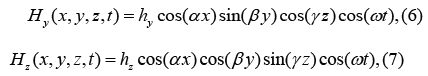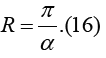• Submissions# To the Rationale for Homeopathy

Solomon I Khmelnik*

Department of Fuel-Free Energy, Israel

*Corresponding author:Solomon I Khmelnik, Department of Fuel-Free Energy, Israel

Submission: January 13, 2020;Published: February 7, 2020Volume1 Issue5
February, 2020

#### Abstract

It is shown that in the water molecules there can exist a bulk standing electromagnetic wave with a frequency of the order of 1017 s-1. This wave can be modulated by the frequency of natural vibrations of the substances dissolved in it. Then it is shown that on the basis of these facts the main features of homeopathy can be explained, including lack of influence of impurities and enhancement of the therapeutic effect when dilution.

#### Introduction

The theoretical rationale for homeopathy does not correspond to scientific ideas about the functioning of organisms. Therefore, a large number of professional medical and general scientific organizations openly express a negative attitude towards homeopathy. The same organizations point to the lack of evidence of the effectiveness of homeopathy. However, homeopathy exists precisely because many see this evidence. The following is an attempt to find a rigorous scientific justification for homeopathy.
First of all, we note some characteristic features of homeopathy.
A. The healing effects of the drug and the drug solution are the same.
B. Trivial calculations show that in drugs with high dilution, the probability of having at least one molecule of the active substance is close to zero. However, the therapeutic effect remains.
C. With a decrease in the concentration of the drug, the therapeutic effect is enhanced. D. The amount of impurities in a homeopathic medicine is inevitably greater than that of a medicinal substance. Therefore, particles of impurities, obviously, should have a greater effect than particles of a drug substance. However, this is not observed.
E. Some homeopaths believe that the effect of the drug is due to the fact that “water has a memory” that transfers biological information.
F. There is no generally accepted scientific opinion about the existence of a “memory of water” .
Thus, the question of the justification of homeopathy boils down to the question of the ability of water to preserve the memory about substances previously dissolved in it.

#### Electromagnetic Volumetric Standing Wave

In [2,3] it was shown (as a consequence of solving Maxwell’s equations) that in a limited volume of vacuum there can exist an electromagnetic standing wave. This volume may have a variety of shapes. In a Cartesian coordinate system, the solution has the formwhere Eγ, EΦ, Ez are the electric intensities, Hγ, HΦ, Hz are the magnetic intensities, ex, ey ez hx, hy hz are the constant amplitudes of the intensities, α ,β ,γ are the constants, ω is the frequency. These quantities are related by the following equations:For cube are parameters

α = β =γ .(15)

The length of the half-edge of the cube is defined asThen the formula for frequency in vacuum takes the formwhere c is the speed of light in vacuum.

A standing wave is not emitted through the faces of the cube and, in the absence of external energy flows, such a wave retains its energy, frequency and shape of volume. This electromagnetic wave can be modulated by a lower frequency. In this case, this volume turns into a keeper of energy and information. In [2-4], well-known experiments and natural phenomena are considered, which serve as proof of the existence of such a keeper.

#### Water Keeper of Information

We assume that the water molecule is the volume that stores the standing wave. In this case, the standing wave is stored in the space between the oxygen and hydrogen atoms, i.e. in a vacuum. Therefore, we can apply formula (17) to determine the frequency of an electromagnetic wave in a water molecule. The gap between the oxygen and hydrogen atoms, where a standing wave can pulsate, has a size of 10−10mmsee Figure 1. Consequently, for her

R ≈ 5.10−11m.(18)

Figure 1:At a known speed of light in vacuum c ≈ 3.108 from (17, 18) we find the frequency of the electromagnetic field in the water molecule:The frequency f is related to this cyclic frequency ω by the relation

ω = 2π f ,(20)

where do we findWe define wavelengthThus, the wavelength (22) and the radius of the region of existence of the standing wave (18) are related by a relationship of the form:This means that the region of existence of a standing wave increases with increasing wavelength or with decreasing frequency. The own frequency of drug molecules is within

L = (1013 ÷1014 )Hz(24)

see , which is much lower than the frequency (21). Therefore, the frequencies of drugs can modulate the frequency of the electromagnetic field in a water molecule. We also note that the region of existence of a standing wave with a frequency of a drug substance is approximately 107 times greater than the region of a standing wave in a water molecule-compare (21) and (24).

It is known that electromagnetic radiation interacts with the molecules of a substance, causing radiation or absorption of electromagnetic radiation by the molecules of a substance at certain frequencies . Therefore, the molecules of a substance can interact through electromagnetic radiation. A multiple enhancement of the effect of such an interaction appears when the eigenfrequencies of the emitting and absorbing molecules coincide. (In this one can see the homeopathic principle of treating of the like by the like, where the similarity is understood in the sense of similarity of frequencies, and not similarity of chemical composition). It must be assumed that it is precisely the frequencies of drugs that are the catalyst that affects diseased organs. Therefore, water molecules that carry “drug frequencies” act in exactly the same way as the drug substances themselves. This explains paragraphs 1.1 and 1.2.

#### The Effect of High Dilution

Initially, subject to precautions in the solution, the number of drug molecules Mo significantly exceeds the number of impurity molecules M1. We write this fact in the following form:

M0 = kM1.(25)

where k≫1. Accordingly, the number of water molecules Vo, bearing the drug frequency, significantly exceeds the number of molecules V1, bearing the frequency of the impurity. We will record this fact in the following form

Vo = kV1 .(26)

It was noted above that the region of existence of an electromagnetic wave with a frequency of a drug substance is approximately 100times greater than the region of a standing wave in a water molecule. Water molecules carrying any frequencies “charge” free molecules with these frequencies. Due to the mobility of water molecules, it can be assumed that all water molecules are charged of various modulating frequencies and the same relation is preserved between them (26). We denoteWhen diluting the solution and maintaining the same volume, the number of molecules Mo and M1 decreases. However, the number of water molecules remains the same and, as before, all water molecules are charged. Therefore, after breeding

q2 > q.(28)

After many dilutions

q2 →∞.(29)

From (28,27,25) it follows that the number of molecules Mo and M1 tends to zero. Consequently, the effect of impurities disappears. This explains paragraph 1.4. Modulation of a standing wave of water molecules by different molecules of the same substance creates a phase difference of electromagnetic waves in different water molecules. The therapeutic effect depends on the total effect of all water molecules. Obviously, the total effect of molecules with different phases is less than the total effect of “in-phase molecules”. Similarly, to the previous one, it can be shown that dilution increases the number of “in-phase molecules”. This explains paragraph 3.

#### Conclusion

It follows from the above that
A. In water molecules, there are always intrinsic electromagnetic waves, which persist in the form of a standing electromagnetic wave of a certain high frequency.
B. This frequency is modulated by the intrinsic frequency of substances dissolved in water. This frequency is much less than the intrinsic frequency of water.
C. Water retains modulated electromagnetic waves in the absence of the substance itself, which created such waves.
D. Water containing modulated electromagnetic waves affects the body in the same way as the substance that created such waves.
Dilution of water with a therapeutic drug dissolved in it increases the therapeutic effect and weakens the effect of impurities.

﻿

We at Crimson Publishing are a group of people with a combined passion for science and research, who wants to bring to the world a unified platform where all scientific know-how is available read more...